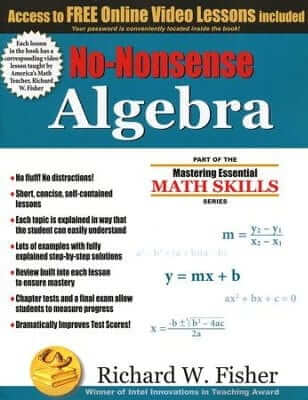# No-Nonsense Algebra

\$38.95

No-Nonsense Algebra uses short, concise lessons to explain each topic in Algebra I in a way that is easy to understand. Multiple examples with fully explained step-by-step solutions, ample reviews built into each of the lessons, and chapter tests help students measure their progress and improve their test scores. Each lesson in the book has a corresponding online video lesson taught by award-winning math teacher and author Richard W. Fisher.

• Chapter 1 Necessary Tools for Algebra
• Chapter 2 Solving Equations
• Chapter 3 Graphing and Analyzing Linear Equations
• Chapter 4 Solving and Graphing Inequalities
• Chapter 5 Systems of Linear Equations and Inequalities
• Chapter 6 Polynomials
• Chapter 7 Rational Expressions (Algebraic Fractions)
• Chapter 8 Radical Expressions and Geometry
• Chapter 10 Algebra Word Problems

Also included:

• A “How to Use This Book” Section
• Chapter reviews
• Final Exam
• Solutions for all Problems
• Glossary
• Resource Center

Each Easy-To-Understand Lesson Includes:

• Each topic is clearly explained in a way that the student can easily understand.
• Examples with simple step-by-step solutions
• Written exercises
• A short Review section that ensures that the student will remember what has been learned